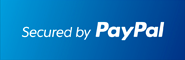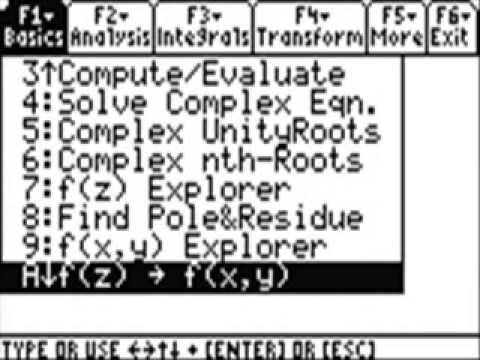** New: ECONOMICS , DIFFERENTIAL EQUATIONS **         ** SPECIALS for bulk apps **         ** Bestsellers: CALCULUS , PHYSICS , CHEM , STOCKS & PORTFOLIOS , ALGEBRA **# #1 Complex Analysis Solver for the TI-89 Calculator

## Solve Complex Analysis problems Step by Step.

### Works With TI92 Plus TI89 TI89 Titanium Voyage 200At the end of the PayPal checkout, you will be sent an email containing your key and software.Description• Complex Numbers Step by Step: Add, Subtract, Multiply, Divide, Exponentiation, Convert Cartesian to Polar (Exponential) Coordinates, Polar to Cartesian Coordinates, Read Tutorial with Examples. Graph Complex Numbers.
• Perform DeMoivre Theorem Step by Step
• Compute , Evaluate expressions involving Complex Numbers, Take the Square Root (Step by Step) , Find the Conjugate, Compute Arg(z), Modulus(z)
• Solve any Complex Equation
• Read Basics on Complex Analysis and Identities involving Trigonometric , Logarithmic, Exponential and Polynomial Functions, DeMoivre Theorem
• f(z) and f(x,y) Step by Step Explorer finds zeros, conjugates, divergence, potential, gradient, curl, expands or factors expressions.
• Compute Roots of Unity and Complex n-th Roots Step by Step
• Find Poles and Residuals of Functions Step by Step.
• ANALYSIS: Find Limits and Derivatives Step by Step, Use Cauchy-Riemann Equations to check if Function is Analytic /Holomorph Step by Step.
• Check if Function is Harmonic, Find Harmonic Conjugate Function (Step by Step). Find Function f(x,y) given its real part u(x,y) .
• INTEGRALS: Find Indefinite Integrals Step by Step. Compute Definite Integrals. Read Integration Rules.
• Compute Curve Integrals along a path , Compute Contour Integrals using Function's Residuals (Step by Step).
• FURTHER: Find Taylor Series and its Interval and Radius of Convergence. Determine its Errors. Check any Series for Convergence using Alternating Series Test., Geometric Series Test, the Integral Test. Find the Sum of any converging Series.
• Perform Partial Fraction Decomposition Step by Step incl. the Cover up Method.
• Read Theorems on Analytic Functions (Morera, Cauchy , etc )
• Read Definition of Continuity, Properties and Rules of Limits, Rules of to find Derivatives.
• Read the Derivatives and Integrals of Power, Trig, Exponential and Logarithm Functions.